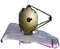# NIRCam Point Spread FunctionsSimulated JWST NIRCam point spread functions (PSFs) in each filter have predicted FWHM between 0.03" and 0.16" assuming nominal mirror wavefront errors.

On this page

At wavelengths λ > μm, JWST obtains diffraction-limited imaging with a Strehl ratio = 0.8 and point spread function (PSF) FWHM of ~λ/D radians (JWST's D = 6.5 m mirror). The NIRCam detectors achieve Nyquist sampling or better (FWHM > 2 pixels) above 2 µm in the short wavelength (SW) channel (0.6–2.3 µm) and above 4 µm in the long wavelength (LW) channel (2.4–5.0 µm). Below these wavelengths, the PSF is undersampled. PSF sampling may be improved by performing subpixel dithers between exposures.

# Simulated NIRCam PSFs

Expected JWST PSFs may be simulated with WebbPSF. Given a wavefront error budget for the mirror alignment, WebbPSF can create multiple realizations of the PSF in a given NIRCam filter. Each of these realizations assumes various contributions to the wavefront error from several optical components, which cause the PSF shape and orientation to vary slightly.

WebbPSF allows the user to select between a "predicted" optical path difference (OPD) map and a "requirements" OPD map. The figures below show one realization for NIRCam imaging in each filter assuming the (slightly more conservative) "requirements" OPD map.

FITS images of simulated PSFs in each filter for LW and SW channels may be downloaded: PSFs_SW_filters and PSFs_LW_filters

These are a single realization of NIRCam PSF FITS images for all filters simulated by WebbPSF using the "requirements" OPD map. SW (LW) PSFs are centered in the A1 (A5) detector. Each PSF is assumed to be centered within a pixel. Each FITS file contains both an oversampled PSF (oversample = 4) and a version rebinned down onto the detector pixel scale (0.031" and 0.063" in the short and long wavelength channels, respectively). Source spectrum is a G5V star spectrum from the PHOENIX model library.

NOTE: Diffraction spikes are oriented relative to the telescope (V3 Position Angle), not NIRCam (see Figure 2 in the NIRCam Field of View page).

Figures 1 and 2 show the PSF in each filter on a log scale. Figures 3 and 4 zoom in to the PSF cores.NIRCam PSFs in each SW channel filter were simulated by WebbPSF. Each PSF image is 299 × 299 detector pixels (9.27" on a side). All are displayed with the same log scale. PSFs are shown in the detector pixel scale (0.031").NIRCam PSF in each LW channel filter were simulated by WebbPSF. Each PSF image is 299 × 299 detector pixels (18.84" on a side), displayed with the same log scaling as Figure 1. PSFs are shown in the detector pixel scale (0.063").These are zooms into the central 32 × 32 pixels (~1" on a side) of each simulated PSF shown in Figure 1. Each PSF is centered within a pixel, displayed with the same log scaling as Figure 1.These are zooms into the central 32 × 32 pixels (~2" on a side) of each simulated PSF shown in Figure 2. Each PSF is centered within a pixel. Displayed with the same log scaling as Figure 1.

# PSF FWHM

Figure 5 shows the FWHM as a function of the filter pivot wavelength for each simulated PSF. Numerical values for each FWHM, in units of arcsec and pixel, are reported in Table 1. Note: due to severe undersampling below 2 µm (4 µm) in the SW (LW) channel, the oversampled PSFs were used to calculate the FWHM.This figure shows the FWHM measured for each of the PSF simulated by WebbPSF, shown in Figures 1–4. Each point, color-coded by wavelength, corresponds to one of  the 29 filters in Table 1. The PSF is undersampled below 2 µm in the SW channel and below 4 µm in the LW channel. Note: for the extra-wide W2 filters (F150W2 and F322W2), the FWHM appears to be smaller then expected due to the choice of the star spectrum.

Table 1. FWHM values for each PSF, from Figure 5

FilterWavelength
(µm)
PSF FWHM
(arcsec)

PSF FWHM (pixel)

F070W0.7040.0300.987
F090W0.9020.0341.103
F115W1.1540.0401.298
F140M1.4050.0481.553
F150W1.5010.0501.628
F162M1.6270.0551.770
F164N1.6450.0561.801
F150W21.6590.0461.494
F182M1.8450.0621.990
F187N1.8740.0642.060
F200W1.9890.0662.141
F210M2.0950.0712.304
F212N2.1210.0722.341
F250M2.5030.0841.340
F277W2.7620.0911.444
F300M2.9890.1001.585
F322W23.2320.0971.547
F323N3.2370.1081.711
F335M3.3620.1111.760
F356W3.5680.1151.830
F360M3.6240.1201.901
F405N4.0520.1362.165
F410M4.0820.1372.179
F430M4.2810.1452.300
F444W4.4080.1452.302
F460M4.6300.1552.459
F466N4.6540.1582.507
F470N4.7080.1602.535
F480M4.8740.1622.574

# Radial profiles

Figures 6 and 7 show the radial profiles for each simulated PSF. The radial profiles have been normalized to the value of the peak pixels.In these plots of the radial profiles for each SW simulated PSF, the pixel size (0.031") is reported in each panel as a dotted line.In these plots of the radial profiles for each simulated LW PSF, the pixel size (0.063") is reported in each panel as a dotted line.

# Encircled and ensquared energy

Figures 8 and 9 show the encircled energy curves for each simulated PSF. Numerical values for the encircled energy (the fraction of light contained in a circular aperture) and ensquared energy (the fraction of energy falling within a certain number of pixels) for each simulated PSF are provided inn these ACII tables:

Encircled_Energy_SW.txt

Encircled_Energy_LW.txt

Ensquared_Energy_SW.txtIn these encircled energy curves for each SW PSF, the radius (in arcsec) at which the encircled energy is 50% and 80% is reported in the figure and in Table 2.In these encircled energy curves for each LW PSF, the radius (in arcsec) at which the encircled energy is 50% and 80% is reported in the figure and in Table 2.

Table 2. Radius (in arcsec) at which the encircled energy (EE) is 50% and 80%
Filter

radius (EE = 50%)

(arcsec)

radius (EE = 80%)

(arcsec)

F070W0.0680.266
F090W0.0600.232
F115W0.0550.213

F140M

0.0430.187
F150W0.0430.189
F162M0.0440.191
F164N0.0440.192
F150W20.0470.197
F182M0.0460.178
F187N0.0470.175
F200W0.0490.176
F210M0.0510.177
F212N0.0510.177
F250M0.0570.181
F277W0.0610.195
F300M0.0660.205
F322W20.0670.218
F323N0.0710.220
F335M0.0730.225
F356W0.0760.235
F360M0.0770.238
F405N0.0860.263
F410M0.0860.266
F430M0.0900.277
F444W0.0920.283
F460M0.0960.295
F466N0.0980.299
F470N0.0990.302
F480M0.1010.308

# References

WebbPSF

Perrin, M., et al. 2014, Proc SPIE 9143
Updated point spread function simulations for JWST with WebbPSF

Published 27 Sep 2017